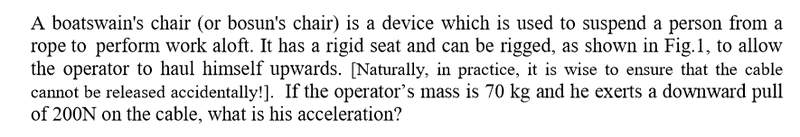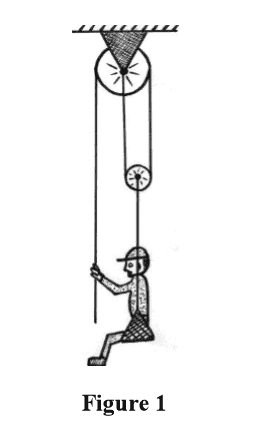# Boatswans Chair pulley Question

## Homework Statement

The question has been screenshotted, along with the given diagram. Basic stuff I know, but I can't seem to get my head around pulley systems. T## Homework Equations

F(net) = m ⋅ a(system)

## The Attempt at a Solution

I understand the rope to be considered inextensible. Also, I understand that the tension must equal the weight of the man & chair, but beyond that I don't know how to break it down.

I labelled a reference frame with x being positive down.

## Answers and Replies

gneill
Mentor
Hi Ryansf98,

Welcome to Physics Forums!

I understand that the tension must equal the weight of the man & chair
What leads you to that conclusion?

Have you made a free body diagram of the man&chair?

Hi Ryansf98,

Welcome to Physics Forums!

What leads you to that conclusion?

Have you made a free body diagram of the man&chair?

I just read that over and now realise it doesn't make sense, as equal tension & weight would mean an acceleration of 0m/s^2 wouldn't it? What I meant was that tension must be equal throughout the rope.

gneill
Mentor
I just read that over and now realise it doesn't make sense, as equal tension & weight would mean an acceleration of 0m/s^2 wouldn't it? What I meant was that tension must be equal throughout the rope.
Okay. Draw the resulting free body diagram for the man&chair.

Hi Ryansf98,

Welcome to Physics Forums!

Thank you by the way! I've used it for sometime looking at existing threads, but I couldn't find an existing problem like this one so thought it'd be a good time to start my own account!

Okay. Draw the resulting free body diagram for the man&chair.

I done that, labelling F(down) as 200N + mg, => F(down total) = 887N.

Regarding the tension, I labelled T moving up from the man to the smaller pulley. Would that then split into 1/2T either way up the pulley, or would it remain T?

I also labelled T pointing up on the rope which the man pulls down on.

Okay. Draw the resulting free body diagram for the man&chair.

I realise I jumped a gun a little there as you only suggested a free body diagram for the man and chair. For this, I labelled mg down and T up towards the smaller pulley. Would I in fact insert 200 N here also?

gneill
Mentor
I realise I jumped a gun a little there as you only suggested a free body diagram for the man and chair. For this, I labelled mg down and T up towards the smaller pulley. Would I in fact insert 200 N here also?
How many ropes attach to the man&chair? If each has a tension T, what's the net force upwards?

How many ropes attach to the man&chair? If each has a tension T, what's the net force upwards?

One rope attached directly to the man and chair, therefore T up? Also, would 200N be T, due to Newtons 3rd Law?

Therefore, Net Force Up = 200N?

How many ropes attach to the man&chair? If each has a tension T, what's the net force upwards?

In fact, there are 3 ropes above the man and pulley, therefore F(up) = 3T.

T = 200N as the man exerts this force down the rope and so the rope exerts this force up on him.

That means that F(net of the system) = mg - 3T, => 686.7 - (3*200) = 86.7N downwards.

F = ma, a= 86.7/70, => a = 1.24 m/s^2?

gneill
Mentor
One rope attached directly to the man and chair, therefore T up? Also, would 200N be T, due to Newtons 3rd Law?

Therefore, Net Force Up = 200N?
No, there are two ropes connected to the man and chair: One he holds directly, one connected to the chair.

The one he holds has a tension T = 200 N. What's the tension in the other rope? (consider a FBD of the small pulley).

gneill
Mentor
In fact, there are 3 ropes above the man and pulley, therefore F(up) = 3T.

T = 200N as the man exerts this force down the rope and so the rope exerts this force up on him.

That means that F(net of the system) = mg - 3T, => 686.7 - (3*200) = 86.7N downwards.

F = ma, a= 86.7/70, => a = 1.24 m/2^2?
Yes! That's much better!

Yes! That's much better!

So generally no matter what, each rope has a tension T throughout the system, even if the rope reaches a pulley and looks to go into 2 separate ropes?

Thanks for the help!!

gneill
Mentor
So generally no matter what, each rope has a tension T throughout the system, even if the rope reaches a pulley and looks to go into 2 separate ropes?
If the pulleys are massless and frictionless, then continuous ropes have the same tension. When separate ropes meet at a pulley, their tensions sum (vector sum).

If the pulleys are massless and frictionless, then continuous ropes have the same tension. When separate ropes meet at a pulley, their tensions sum (vector sum).

Makes sense. Again thanks for your help!